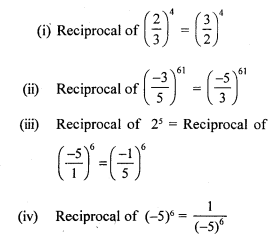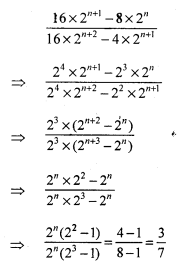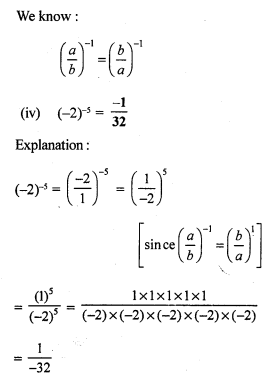# RS Aggarwal Class 7 Solutions Chapter 5 Exponents CCE Test Paper

In this chapter, we provide RS Aggarwal Solutions for Class 7 Chapter 5 Exponents CCE Test Paper for English medium students, Which will very helpful for every student in their exams. Students can download the latest RS Aggarwal Solutions for Class 7 Chapter 5 Exponents CCE Test Paper Maths pdf, free RS Aggarwal Solutions Class 7 Chapter 5 Exponents CCE Test Paper Maths book pdf download. Now you will get step by step solution to each question.

### RS Aggarwal Solutions for Class 7 Chapter 5 Exponents CCE Test Paper Download PDF

Question 1.
Solution:Question 2.
Solution:
Let the required number be xQuestion 3.
Solution:
Let the required number be x.
(-20)-1 ÷ x = (-10)-1Question 4.
Solution:
(i) 2000000 = 2.000000 x 106 [Since the decimal point is moved 6 to the left]
= 2 x 106
(ii) 6.4 x 105 = 6.4 x 100000 = 640000

Question 5.
Solution:
We have :Question 6.
Solution:
We have :Mark (✓) against the correct answer in each of the following :
Question 7.
Solution:Question 8.
Solution:Question 9.
Solution:Question 10.
Solution:Question 11.
Solution:Question 12.
Solution:
(c) 3.263 x 105
A given number is said to be in standard form if it can be expressed as
k x 10n, where k is a real number such that 1 < k < 10 and n is a positive integer.
For example : 3.263 x 105

Question 13.
Solution:Question 14.
Solution:
(i) True
645 = 6.45 x 102
[Since the decimal point is moved 2 places to the left]
(ii) False
27000 = 2.7 x 104
[Since the decimal point is moved 4 places to the left]
(iii) False
(3° + 4° + 5°) = 1
(iv) False
Reciprocal of 56 = Reciprocal ofAll Chapter RS Aggarwal Solutions For Class 7 Maths

—————————————————————————–

All Subject NCERT Exemplar Problems Solutions For Class 7

All Subject NCERT Solutions For Class 7

*************************************************

I think you got complete solutions for this chapter. If You have any queries regarding this chapter, please comment on the below section our subject teacher will answer you. We tried our best to give complete solutions so you got good marks in your exam.

If these solutions have helped you, you can also share rsaggarwalsolutions.in to your friends.